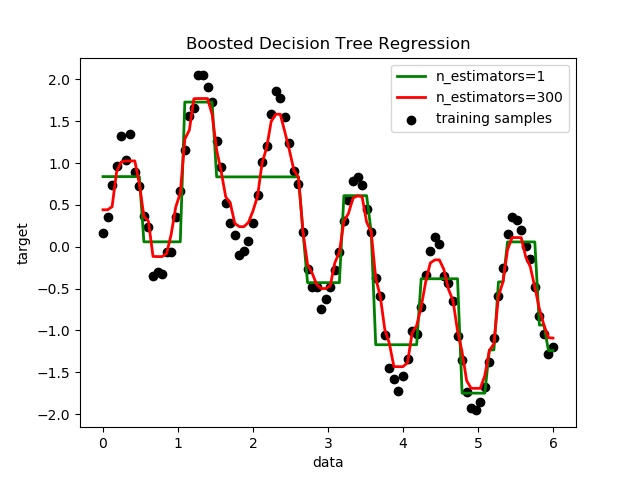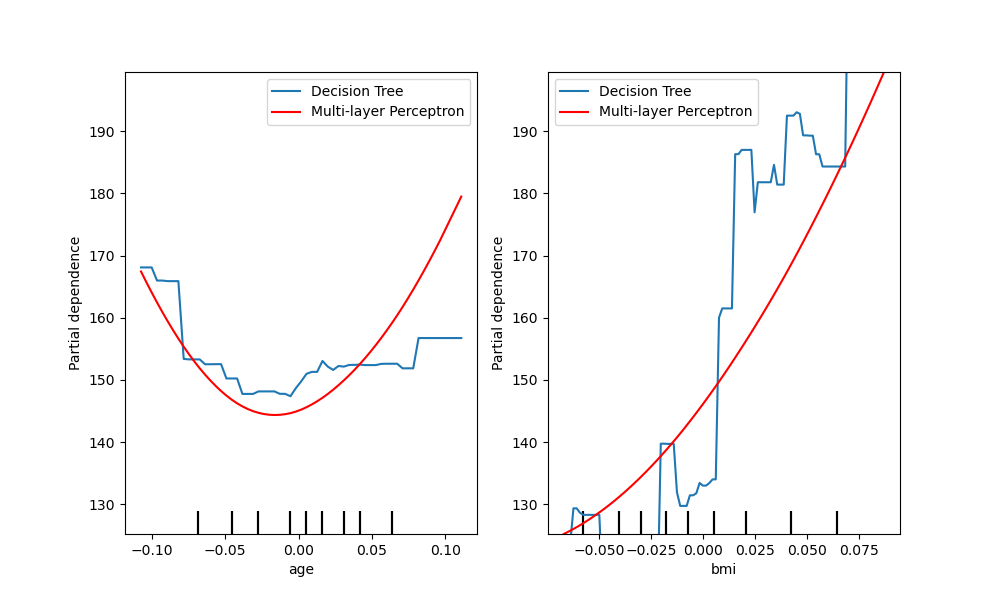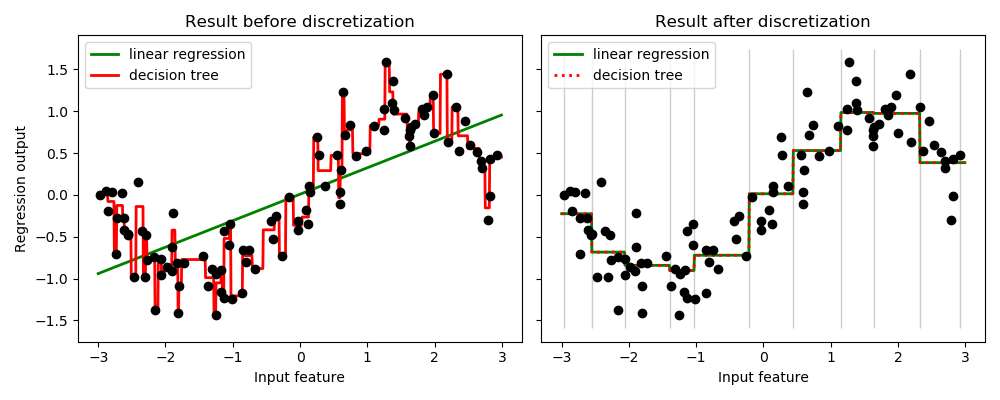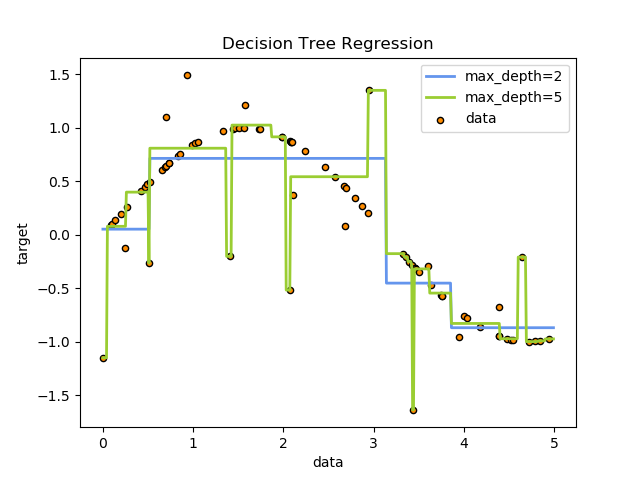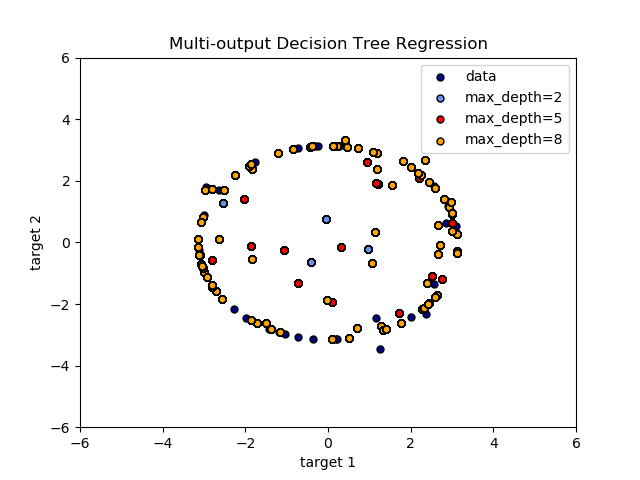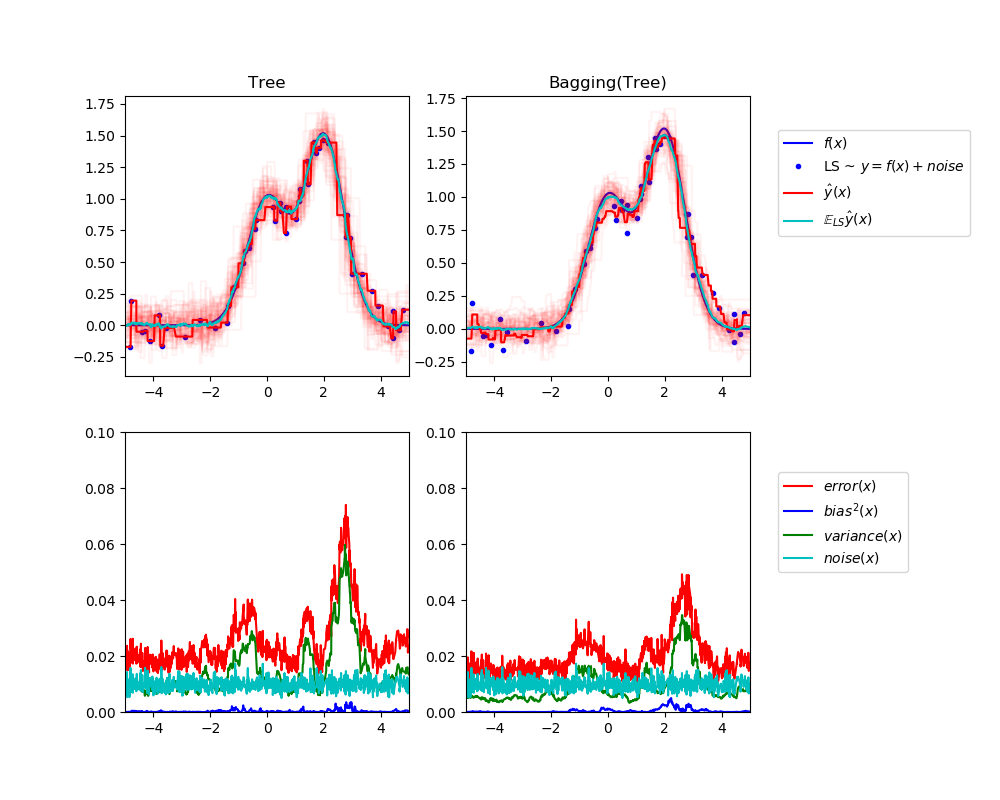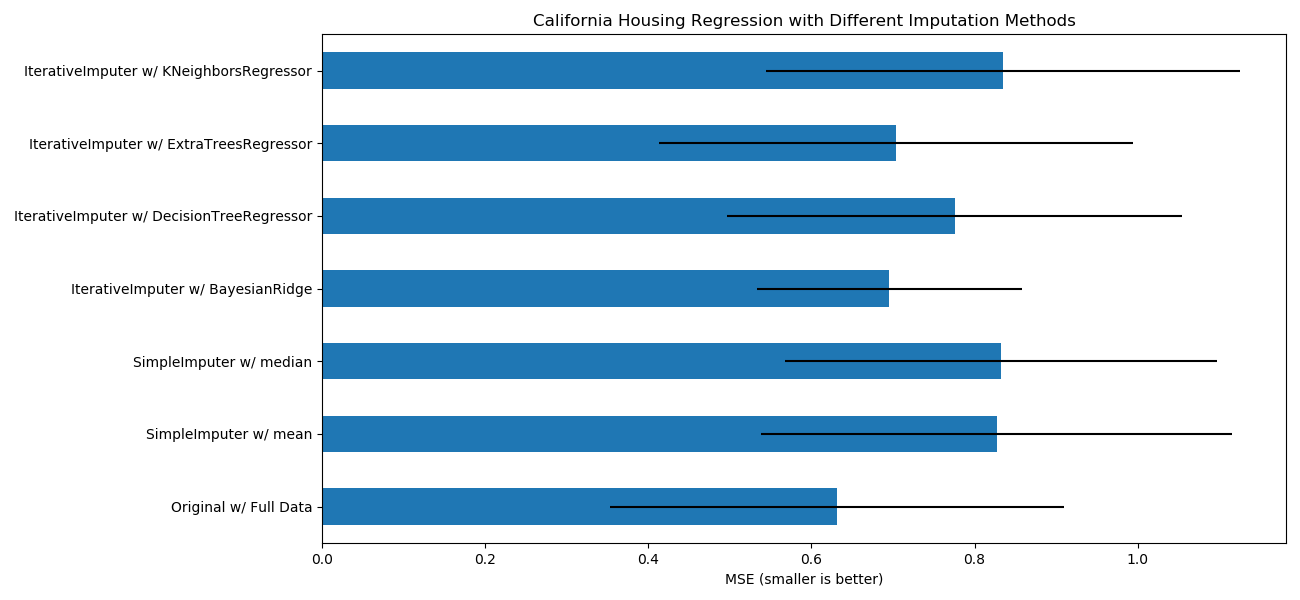# sklearn.tree.DecisionTreeRegressor¶

class sklearn.tree.DecisionTreeRegressor(*, criterion='mse', splitter='best', max_depth=None, min_samples_split=2, min_samples_leaf=1, min_weight_fraction_leaf=0.0, max_features=None, random_state=None, max_leaf_nodes=None, min_impurity_decrease=0.0, min_impurity_split=None, presort='deprecated', ccp_alpha=0.0)

[源码]

criterion {“mse”, “friedman_mse”, “mae”}, default=”mse”

splitter {“best”, “random”}, default=”best”

max_depth int, default=None

min_samples_split int or float, default=2

- 如果为int，则认为min_samples_split是最小值。
- 如果为float，min_samples_split则为分数， ceil(min_samples_split * n_samples)是每个拆分的最小样本数。

min_samples_leaf int or float, default=1

min_weight_fraction_leaf float, default=0.0

max_features int, float or {“auto”, “sqrt”, “log2”}, default=None

- 如果为int，则max_features在每个分割处考虑特征。
- 如果为float，max_features则为小数，并且int(max_features * n_features)是在每次拆分时考虑要素。
- 如果为“auto”，则为max_features=n_features
- 如果是“ sqrt”，则max_features=sqrt(n_features)
- 如果为“ log2”，则为max_features=log2(n_features)
- 如果为”None“，则max_features=n_features

random_state int, RandomState instance, default=None

max_leaf_nodes int, default=None

min_impurity_decrease float, default=0.0

N_t / N * (impurity - N_t_R / N_t * right_impurity N_t_L / N_t * left_impurity)

NN_tN_t_RN_t_L都指的是加权和，如果sample_weight可以通过。

min_impurity_split float, (default=0)

presort deprecated, default=’deprecated’

从0.22版开始不推荐使用。
ccp_alpha non-negative float, default=0.0

0.22版中的新功能。

feature_importances_ ndarray of shape (n_features,)

max_features_ int
max_features的推断值。
n_features_ int
fit执行时的特征数量。
n_outputs_ int
fit执行时的输出数量。
tree_ Tree

DecisionTreeClassifier

1 https://en.wikipedia.org/wiki/Decision_tree_learning

2 L. Breiman, J. Friedman, R. Olshen, and C. Stone, “Classification and Regression Trees”, Wadsworth, Belmont, CA, 1984.

3 T. Hastie, R. Tibshirani and J. Friedman. “Elements of Statistical Learning”, Springer, 2009.

4 L. Breiman, and A. Cutler, “Random Forests”, https://www.stat.berkeley.edu/~breiman/RandomForests/cc_home.htm

>>> from sklearn.datasets import load_diabetes>>> from sklearn.model_selection import cross_val_score>>> from sklearn.tree import DecisionTreeRegressor>>> X, y = load_diabetes(return_X_y=True)>>> regressor = DecisionTreeRegressor(random_state=0)>>> cross_val_score(regressor, X, y, cv=10)...                    # doctest: +SKIP...array([-0.39..., -0.46...,  0.02...,  0.06..., -0.50...,       0.16...,  0.11..., -0.73..., -0.30..., -0.00...])

apply(X[, check_input]) 返回每个样本被预测为的叶子的索引。
cost_complexity_pruning_path(X, y[, …]) 在最小化成本复杂性修剪期间计算修剪路径。
decision_path(X[, check_input]) 返回树中的决策路径。
fit(X, y[, sample_weight, check_input, …]) 根据训练集（X，y）构建决策树回归器。
get_depth() 返回决策树的深度。
get_n_leaves() 返回决策树的叶子数。
get_params([deep]) 获取此估计量的参数。
predict(X[, check_input]) 预测X的类或回归值。
score(X, y[, sample_weight]) 返回预测的确定系数R ^ 2。
set_params(**params) 设置此估算器的参数。
__init__(*, criterion='mse', splitter='best', max_depth=None, min_samples_split=2, min_samples_leaf=1, min_weight_fraction_leaf=0.0, max_features=None, random_state=None, max_leaf_nodes=None, min_impurity_decrease=0.0, min_impurity_split=None, presort='deprecated', ccp_alpha=0.0)

apply(X, check_input=True)

[源码]

0.17版中的新功能。

X {array-like, sparse matrix} of shape (n_samples, n_features)

check_input bool, default=True

X_leaves array-like of shape (n_samples,)

cost_complexity_pruning_path(X, y, sample_weight=None)

[源码]

X {array-like, sparse matrix} of shape (n_samples, n_features)

y array-like of shape (n_samples,) or (n_samples, n_outputs)

sample_weight array-like of shape (n_samples,), default=None

ccp_path Bunch

ccp_alphas：ndarray

impurities：ndarray

decision_path(X, check_input=True)

[源码]

X {array-like, sparse matrix} of shape (n_samples, n_features)

check_input bool, default=True

indicator sparse matrix of shape (n_samples, n_nodes)

property feature_importances_

feature_importances_ ndarray of shape (n_features,)

fit(X, y, sample_weight=None, check_input=True, X_idx_sorted=None)

[源码]

X {array-like, sparse matrix} of shape (n_samples, n_features)

y array-like of shape (n_samples,) or (n_samples, n_outputs)

sample_weight array-like of shape (n_samples,), default=None

check_input bool, default=True

X_idx_sorted array-like of shape (n_samples, n_features), default=None

self DecisionTreeRegressor

get_depth()

[源码]

self.tree_.max_depth int

get_n_leaves()

[源码]

self.tree_.n_leaves int

get_params(deep=True)

[源码]

deep bool, default=True

params mapping of string to any

predict(X, check_input=True)

[源码]

X {array-like, sparse matrix} of shape (n_samples, n_features)

check_input bool, default=True

y array-like of shape (n_samples,) or (n_samples, n_outputs)

score(X, y, sample_weight=None)

[源码]

X array-like of shape (n_samples, n_features)

y array-like of shape (n_samples,) or (n_samples, n_outputs)
X的真实值。
sample_weight array-like of shape (n_samples,), default=None

score float
self.predict(X) wrt. y的R^2

set_params(**params)

[源码]

**params dict

self object

## sklearn.tree.DecisionTreeRegressor使用实例¶